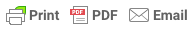Sorry, you do not have permission to ask a question, You must login to ask question. Become VIP Member

Do you need to remove the ads? Become VIP Member

If the chain that is employed for measuring length is not equal to the true length, then the length measured won’t be correct. This measurement hence requires correction.

A chain that is very long, gives a distance that is lesser in value. This means the error is negative and the correction is positive. A chain that is short, gives larger distance value. In this case, the error is positive and the correction is negative.

If,

L = True length or designated length of the chain or tape

L’ = Incorrect or Actual Length of the chain or tape used

The correction for length, area and volume can be given by the following formulas.

## 1. Correction to Measured Length

If ‘l’ is the actual or true length of the line and l’ is the measured length of the line then,

True length of the line = Measured length x [L’/L]& Ad Free!

GET VIP MEMBERSHIP NOW!

## 2. Correction to Area

If ‘A’ is the actual or the true area of the ground and A’ is the measured or the calculated area of the ground, then

True Area = Measured Area x Square of [L’/L]

Also,

L’/L = [(L +dL) / L] = 1 + (dL /L) Eq.3

dL = Error in the length of chain;

Let dL/L =e;

Eq.3 becomes,

L’/L = 1+e

Therefore,

A= (1+e)(1+e) . A’

A=[ 1+2e+ (e.e)] A’

If e is very small, then

## 3. Correction to Volume

If V is the actual or the true volume and V’ is the measured or the computed volume, then

True volume = Measured Volume x cube of [L’/L]

Also,

L’/L = [(L +dL) / L] = 1 + (dL /L) Eq.5

dL = Error in the length of chain;

Let dL/L =e; Therefore Eq.5. becomes

L’/L = 1+e

Eq.5 becomes,

V = (1+e)(1+e)(1+e).V;

By solving,

### V = (1+3e)V’

Also Read: Errors in Chaining -Causes and Types

EDITOR